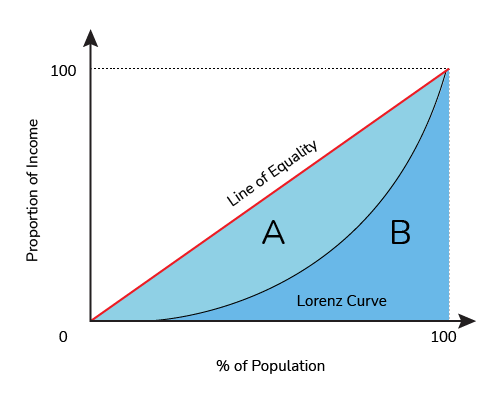# Gini Coefficient Calculator

Created by Wei Bin Loo
Reviewed by Anna Szczepanek, PhD and Steven Wooding
Last updated: Jun 05, 2023

With this Gini coefficient calculator, we aim to help you to measure the income equality of a region or country. The Gini coefficient enables you to understand how income is distributed within the population of a country or a region.

We have written this article to help you understand what the Gini coefficient is and how to calculate the Gini coefficient. We will also demonstrate some Gini coefficients by country calculations to help you understand the income distribution concept.

💡 You might also find our disposable income calculator useful when analyzing incomes.

## What is Gini coefficient? The Gini coefficient definition

The Gini coefficient, also called the Gini index, is a statical metric used to measure wealth inequality within a nation. It ranges from 0 to 1, with 1 indicating a complete inequality of wealth. A Gini coefficient of 0 means that the wealth is evenly distributed among all citizens, meaning complete equality in income. On the other hand, 1 means that one person owns all the wealth within the country.

Developed by Corrado Gini in 1912, the Gini coefficient by country is used by the World Bank and the UN in multiple functions. Most governments use this metric to keep track of the income distribution of their countries too.

## How to calculate Gini coefficient? – Gini coefficient formula

You can calculate the Gini coefficient using the Lorenz curve (see figure below). Each percentile of the population and income is populated on the graph. A 45-degree strategy line is called the line of equality. This is because it means that the top 10% of the population receives 10% of the income while the bottom 10% also receives 10% of the income.Now, let's look at how to calculate the Gini coefficient using the Lorenz curve. There are two areas on the Lorenz Curve. Let's called the area between the Lorenz curve and the line of equality area A and the area under the Lorenz curve area B. Look at the example below:

• Graph: Lorenz curve.
• Area A: 1,200 units².
• Area B: 3,800 units².

You can calculate the Gini coefficient in three steps:

1. Determine the area above the Lorenz curve and below the line of equality (A).

The first step is to calculate the area A, which is the area above the Lorenz curve and below the line of equality. For our example, this area is 1,200 units².

Please check out our triangle area calculator and area of a rectangle calculator to help with this task.

2. Determine the area below the Lorenz curve (B).

Next, we need to calculate the area below the Lorenz curve, area B. The area B in our example is 3,800 units².

3. Calculate the Gini coefficient formula.

The last step is to calculate the Gini coefficient using the formula below:

Gini coefficient = A / (A + B)

In this example, the Gini coefficient is:

1,200 / (1,200 + 3,800) = 0.24.

## FAQ

### What is the US Gini coefficient?

The US Gini coefficient was 0.49 in 2021. This number is the same as Lithuania. This means that even though the US has a better quality of life, income equality is as bad as it is in Lithuania.

### Can the Gini coefficient be negative?

No, the Gini coefficient can never be negative. This is because the numerator and denominator of the Gini coefficient are positive numbers.

### How can I calculate the Gini coefficient?

You can calculate the Gini coefficient in three steps:

1. Determine the area above the Lorenz curve and below the line of equality (A).

2. Determine the area below the Lorenz curve (B).

3. Apply the Gini coefficient formula:

Gini coefficient = A / (A + B)

### What is the Gini coefficient if the Lorenz curve is a straight line?

The Gini coefficient will be 0. This means the country's income is evenly distributed among all its citizens.

Wei Bin Loo
Area A
unit²
Area B
unit²
Gini coefficientPeople also viewed…

### Black Friday

How to get best deals on Black Friday? The struggle is real, let us help you with this Black Friday calculator!

### Break even

Break-even calculator shows you how much revenue your business needs to make in order to cover all costs.

### Fisher effect

Our Fisher Effect calculator helps you to understand the relationship between expected inflation and interest rates.

### Lost socks

Socks Loss Index estimates the chance of losing a sock in the laundry.